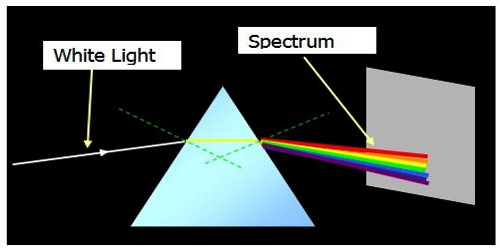# Cause of Formation of Spectrum

Cause of Formation of Spectrum

We know, after refraction of a ray of light through a prism it bends towards the base of the prism. How much a ray will bend depends on the refractive index of the material of the prism, angle of incidence and the angle of the prism. Again, if different rays are incident at the same angle of incidence on a prism, then how much a ray will bend will depend, besides the refractive index of the material of the prism, on the color of light. Different colors of light have different wavelengths. The wavelengths of red light more, approximately 8000 Å, so its angle of deviation is less. On the other hand, the wavelength of violet light is less, approximately 4000 Å, so its angle of deviation is more while passing through a prism.

Furthermore, lights of different colors have different irrefrangibility. From the above explanations, we can make the following two conclusions about the dispersion or cause for the formation of the spectrum –

(1) Since the angle of deviation for different colors of the light is different due to the difference of wavelength, so the spectrum is formed.Fig: Refractive Index versus Wavelength

(2) The seven fundamental colors, that exist in white light have different; refractive indices of the medium, hence spectrum is formed. In the figure, a graph of refractive index versus wavelength has been shown. It is seen from the graph that the light having a higher wavelength, has a lower refractive index, so it bends less. For this reason, the refraction of red light is on the other hand, the wavelength of violet light is less, hence its refractive index more. As a result, the refraction of violet light is more, i.e. it bends more.